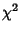## Fisher's Theorem

Letbe a sum of squares ofindependent normal standardized variates, and supposewhereis a quadratic form in the, distributed as Chi-Squared withDegrees of Freedom. Thenis distributed aswithDegrees of Freedom and is independent of. The converse of this theorem is known as Cochran's Theorem.